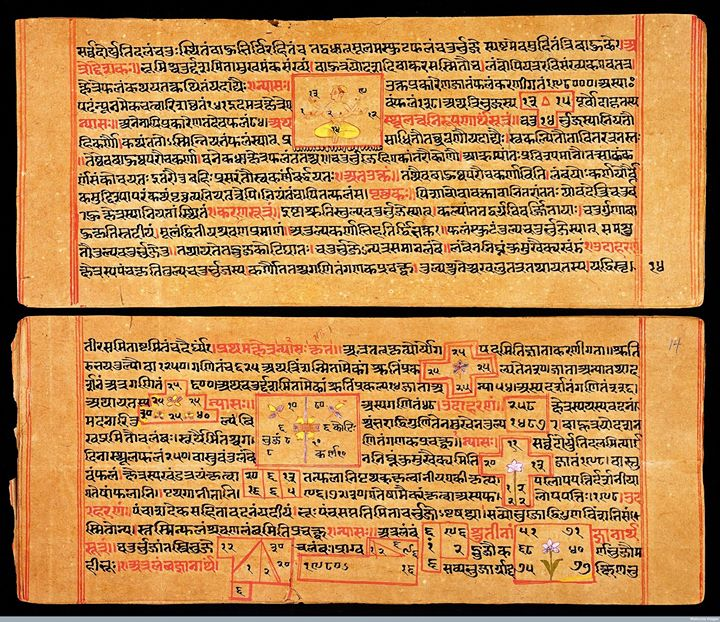Posted on: 6 January 2015

Digital Rare Book:
Bhaskara's BIJA GANITA or The Algebra of the Hindus
By Edward Strachey
Of The East India Company's Bengal Civil Service

http://bit.ly/13Xcxdn

http://bit.ly/1yzPEdA

Bhaskara (1114 – 1185),also known as Bhaskara II and Bhaskara Ach?rya ("Bhaskara the teacher")) was an Indian mathematician and astronomer. He was born near Bijjada Bida (in present day Bijapur district, Karnataka state, South India) into the Deshastha Brahmin family. Bhaskara was head of an astronomical observatory at Ujjain, the leading mathematical centre of ancient India. His predecessors in this post had included both the noted Indian mathematician Brahmagupta (598–c. 665) and Varahamihira. He lived in the Sahyadri region.

Bhaskara and his works represent a significant contribution to mathematical and astronomical knowledge in the 12th century. His main works were the Lilavati (dealing with arithmetic), Bijaganita (Algebra) and Siddhanta Shiromani (written in 1150) which consists of two parts: Goladhyaya (sphere) and Grahaganita (mathematics of the planets).

Some of Bhaskara's contributions to mathematics include the following:
- A proof of the Pythagorean theorem by calculating the same area in two different ways and then canceling out terms to get a˛ + b˛ = c˛.
- In Lilavati, solutions of quadratic, cubic and quartic indeterminate equations.
- Solutions of indeterminate quadratic equations (of the type ax˛ + b = y˛).
- Integer solutions of linear and quadratic indeterminate equations (Kuttaka). -The rules he gives are (in effect) the same as those given by the Renaissance European mathematicians of the 17th century
- A cyclic Chakravala method for solving indeterminate equations of the form ax˛ + bx + c = y. The solution to this equation was traditionally attributed to William Brouncker in 1657, though his method was more difficult than the chakravala method.
- His method for finding the solutions of the problem x˛ ? ny˛ = 1 (so-called "Pell's equation") is of considerable interest and importance.
- Solutions of Diophantine equations of the second order, such as 61x˛ + 1 = y˛. This very equation was posed as a problem in 1657 by the French mathematician Pierre de Fermat, but its solution was unknown in Europe until the time of Euler in the 18th century.
- Solved quadratic equations with more than one unknown, and found negative and irrational solutions.
- Preliminary concept of mathematical analysis.
- Preliminary concept of infinitesimal calculus, along with notable contributions towards integral calculus.
- Conceived differential calculus, after discovering the derivative and differential coefficient.
- Stated Rolle's theorem, a special case of one of the most important theorems in analysis, the mean value theorem.
- Traces of the general mean value theorem are also found in his works.
- Calculated the derivatives of trigonometric functions and formulae.
- In Siddhanta Shiromani, Bhaskara developed spherical trigonometry along with a number of other trigonometric results.
- Wiki

Image: